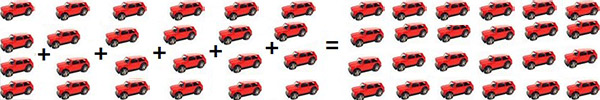# Multiplication as Repeated Addition

#### Complete Python Prime Pack

9 Courses     2 eBooks

#### Artificial Intelligence & Machine Learning Prime Pack

6 Courses     1 eBooks

#### Java Prime Pack

9 Courses     2 eBooks

Let us consider an example to understand multiplication as a repeated addition operation. Suppose there are some toy cars divided into 6 groups each having 4 toy cars. The total number of toy cars can be found by adding 4 repeatedly 6 times as shown below.

4 + 4 + 4 + 4 + 4 + 4 = 24The same result is also obtained by multiplication operation. Since 4 is being repeatedly added take 4 and multiply it with the number of groups which is 6.

So 4 × 6 = 24 (Read as 4 times 6 equals 24)

Here 4 and 6 care called as factors and the resulting number is called as product.

The way multiplication is related to repeated addition can be explained in this way, i.e. multiplying a × b is the same as adding a repeatedly b number of times.

For example, for objects arranged in 4 rows and 5 columns (20 in all);

5 + 5 + 5 + 5 and 4 × 5 represents the total number of objects.

When you multiply, you add equal groups together to find the total.Write the number of keys that you see. Write it as both an addition and multiplication problem

2 + 2 + 2 + 2 =

4 × 2 =

### Solution

Step 1:

We see 2 keys in each group. There are 4 groups.

To simplify, there are 4 groups of 2 keys or

2 + 2 + 2 + 2 = 8

Step 2:

This can also be written as a multiplication problem.

4 groups and each group has 2 keys so we can multiply

4 × 2 = 8

Step 3:

So,

2 + 2 + 2 + 2 = 8

4 × 2 = 8

Rewrite the following repeated addition as a multiplication sentence

2 + 2 + 2 + 2 +2 + 2 = 12

### Solution

Step 1:

Here 2 is being repeatedly added, so first write a 2. Then we count the number of times it is being added. This is 6 times.

Step 2:

So the multiplication sentence would be

2 × 6 = 12